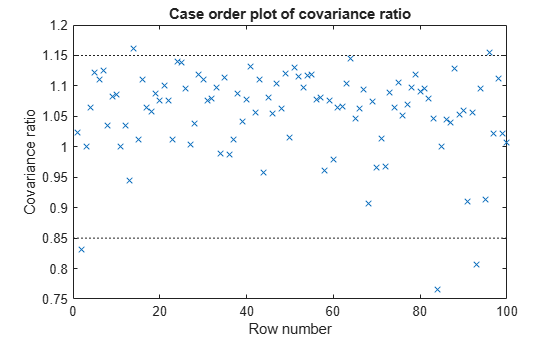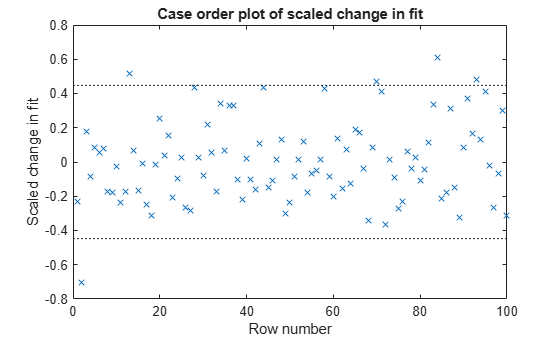Delete-1 Statistics

Delete-1 Change in Covariance (CovRatio)

Purpose

Delete-1 change in covariance (CovRatio) identifies the observations that are influential in the regression fit. An influential observation is one where its exclusion from the model might significantly alter the regression function. Values of CovRatio larger than 1 + 3*p/n or smaller than 1 – 3*p/n indicate influential points, where p is the number of regression coefficients, and n is the number of observations.

Definition

The CovRatio statistic is the ratio of the determinant of the coefficient covariance matrix with observation i deleted to the determinant of the covariance matrix for the full model:

$\text{CovRatio}=\frac{\mathrm{det}\left\{MSE\left(i\right){\left[{X}^{\prime }\left(i\right)X\left(i\right)\right]}^{-1}\right\}}{\mathrm{det}\left[MSE{\left({X}^{\prime }X\right)}^{-1}\right]}.$

CovRatio is an n-by-1 vector in the Diagnostics table of the fitted LinearModel object. Each element is the ratio of the generalized variance of the estimated coefficients when the corresponding element is deleted to the generalized variance of the coefficients using all the data.

How To

After obtaining a fitted model, say, mdl, using fitlm or stepwiselm, you can:

• Display the CovRatio by indexing into the property using dot notation

mdl.Diagnostics.CovRatio

• Plot the delete-1 change in covariance using

plotDiagnostics(mdl,'CovRatio')
For details, see the plotDiagnostics method of the LinearModel class.

Determine Influential Observations Using CovRatio

This example shows how to use the CovRatio statistics to determine the influential points in data. Load the sample data and define the response and predictor variables.

y = hospital.BloodPressure(:,1);
X = double(hospital(:,2:5));

Fit a linear regression model.

mdl = fitlm(X,y);

Plot the CovRatio statistics.

plotDiagnostics(mdl,'CovRatio')For this example, the threshold limits are 1 + 3*5/100 = 1.15 and 1 - 3*5/100 = 0.85. There are a few points beyond the limits, which might be influential points.

Find the observations that are beyond the limits.

find((mdl.Diagnostics.CovRatio)>1.15|(mdl.Diagnostics.CovRatio)<0.85)
ans = 5×1

2
14
84
93
96

Delete-1 Scaled Difference in Coefficient Estimates (Dfbetas)

Purpose

The sign of a delete-1 scaled difference in coefficient estimate (Dfbetas) for coefficient j and observation i indicates whether that observation causes an increase or decrease in the estimate of the regression coefficient. The absolute value of a Dfbetas indicates the magnitude of the difference relative to the estimated standard deviation of the regression coefficient. A Dfbetas value larger than 3/sqrt(n) in absolute value indicates that the observation has a large influence on the corresponding coefficient.

Definition

Dfbetas for coefficient j and observation i is the ratio of the difference in the estimate of coefficient j using all observations and the one obtained by removing observation i, and the standard error of the coefficient estimate obtained by removing observation i. The Dfbetas for coefficient j and observation i is

$Dfbeta{s}_{ij}=\frac{{b}_{j}-{b}_{j\left(i\right)}}{\sqrt{MS{E}_{\left(i\right)}}\left(1-{h}_{ii}\right)},$

where bj is the estimate for coefficient j, bj(i) is the estimate for coefficient j by removing observation i, MSE(i) is the mean squared error of the regression fit by removing observation i, and hii is the leverage value for observation i. Dfbetas is an n-by-p matrix in the Diagnostics table of the fitted LinearModel object. Each cell of Dfbetas corresponds to the Dfbetas value for the corresponding coefficient obtained by removing the corresponding observation.

How To

After obtaining a fitted model, say, mdl, using fitlm or stepwiselm, you can obtain the Dfbetas values as an n-by-p matrix by indexing into the property using dot notation,

mdl.Diagnostics.Dfbetas

Determine Observations Influential on Coefficients Using Dfbetas

This example shows how to determine the observations that have large influence on coefficients using Dfbetas. Load the sample data and define the response and independent variables.

y = hospital.BloodPressure(:,1);
X = double(hospital(:,2:5));

Fit a linear regression model.

mdl = fitlm(X,y);

Find the Dfbetas values that are high in absolute value.

[row,col] = find(abs(mdl.Diagnostics.Dfbetas)>3/sqrt(100));
disp([row col])
2     1
28     1
84     1
93     1
2     2
13     3
84     3
2     4
84     4

Delete-1 Scaled Change in Fitted Values (Dffits)

Purpose

The delete-1 scaled change in fitted values (Dffits) show the influence of each observation on the fitted response values. Dffits values with an absolute value larger than 2*sqrt(p/n) might be influential.

Definition

Dffits for observation i is

${\text{Dffits}}_{i}=s{r}_{i}\sqrt{\frac{{h}_{ii}}{1-{h}_{ii}}},$

where sri is the studentized residual, and hii is the leverage value of the fitted LinearModel object. Dffits is an n-by-1 column vector in the Diagnostics table of the fitted LinearModel object. Each element in Dffits is the change in the fitted value caused by deleting the corresponding observation and scaling by the standard error.

How To

After obtaining a fitted model, say, mdl, using fitlm or stepwiselm, you can:

• Display the Dffits values by indexing into the property using dot notation

mdl.Diagnostics.Dffits

• Plot the delete-1 scaled change in fitted values using

plotDiagnostics(mdl,'Dffits')
For details, see the plotDiagnostics method of the LinearModel class for details.

Determine Observations Influential on Fitted Response Using Dffits

This example shows how to determine the observations that are influential on the fitted response values using Dffits values. Load the sample data and define the response and independent variables.

y = hospital.BloodPressure(:,1);
X = double(hospital(:,2:5));

Fit a linear regression model.

mdl = fitlm(X,y);

Plot the Dffits values.

plotDiagnostics(mdl,'Dffits')The influential threshold limit for the absolute value of Dffits in this example is 2*sqrt(5/100) = 0.45. Again, there are some observations with Dffits values beyond the recommended limits.

Find the Dffits values that are large in absolute value.

find(abs(mdl.Diagnostics.Dffits)>2*sqrt(4/100))
ans = 10×1

2
13
28
44
58
70
71
84
93
95

Delete-1 Variance (S2_i)

Purpose

The delete-1 variance (S2_i) shows how the mean squared error changes when an observation is removed from the data set. You can compare the S2_i values with the value of the mean squared error.

Definition

S2_i is a set of residual variance estimates obtained by deleting each observation in turn. The S2_i value for observation i is

$S2_i=MS{E}_{\left(i\right)}=\frac{\sum _{j\ne i}^{n}{\left[{y}_{j}-{\stackrel{^}{y}}_{j\left(i\right)}\right]}^{2}}{n-p-1},$

where yj is the jth observed response value. S2_i is an n-by-1 vector in the Diagnostics table of the fitted LinearModel object. Each element in S2_i is the mean squared error of the regression obtained by deleting that observation.

How To

After obtaining a fitted model, say, mdl, using fitlm or stepwiselm, you can:

• Display the S2_i vector by indexing into the property using dot notation

mdl.Diagnostics.S2_i

• Plot the delete-1 variance values using

plotDiagnostics(mdl,'S2_i')
For details, see the plotDiagnostics method of the LinearModel class.

Compute and Examine Delete-1 Variance Values

This example shows how to compute and plot S2_i values to examine the change in the mean squared error when an observation is removed from the data. Load the sample data and define the response and independent variables.

y = hospital.BloodPressure(:,1);
X = double(hospital(:,2:5));

Fit a linear regression model.

mdl = fitlm(X,y);

Display the MSE value for the model.

mdl.MSE
ans = 23.1140

Plot the S2_i values.

plotDiagnostics(mdl,'S2_i')This plot makes it easy to compare the S2_i values to the MSE value of 23.114, indicated by the horizontal dashed lines. You can see how deleting one observation changes the error variance.